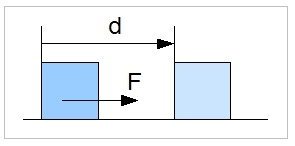# Work, Energy and Power

## Work

Work is defined as:

W = F . d

where:
F : Force (N)
d : Displacement (m)

The amount of work 'W' carried out by a force 'F' is defined by the distance 'd' the working point of the force travels. The amount of work is defined by the force component, which is in the same direction as the displacement.### Explanation

The quantity, work, is utilised to calculate the amount of energy used. Work is a scalar quantity, and can be positive or negative. A positive amount of work tries to accelerate an object, and a negative amount tries to decelerate an object (mechanical physics). It is possible to have a zero amount of work, although the force involved is not equal to zero. For example, when a book is lying on a table, there is a force working on the book (due to gravity). However, the book is not moving, so there is no distance which the force's working point is travelling.

### Examples

An object is lying on a table and is moved 2 metres along it. The manoeuvre required a force of 10 N. The amount of work involved is 2 times 10, equals 20 Nm (or J).

An object is falling down and must be prevented from reaching the ground. An upward force is, therefore, applied. This force generates a negative amount of work, because the force and travel direction are in opposing directions.

## Energy

Energy is defined as:

The ability to do work (in Joules).

### Explanation

Energy is an attribute of a system or object. This means that a system or object contains an amount of energy. This amount of energy can be used to carry out Work.

### Example

A rock with a mass of 1 kg is positioned at a height of 10 metres above the earth's surface. When this rock falls, due to gravity, work is carried out. The amount is F . d = m. g . d  = 1 . 9.8 . 10 = 98 Nm (J), with g the gravitational acceleration. So, the rock at a height of 10 metres above the surface possesses 98J of (potential) energy. This energy is changed into kinetic energy during the rock's fall.

## Conservation of Energy

In brief, the law of the conservation of energy states that energy cannot be created or destroyed; it can only be changed from one form to another, or transferred from one body to another, but the total amount of energy remains constant (the same).

In mechanics, conservation of energy is usually stated as

E = T + V,

where T is kinetic and V potential energy.

### Explanation

The total amount of energy in a closed system stays the same. It can only alter in form. So, when a rock falls, potential energy is transferred into kinetic energy.

### Examples

At what speed does the rock from the example above hit the ground? When the rock falls, all its potential energy becomes kinetic energy. Kinetic energy is defined as: (1/2) . m . V2. This leads to:

98 = (1/2) . m . V2

So, the speed at which the rock hits the ground is: v = sqrt(2 . 98 / m) = sqrt (196) = 14 m/s.

## Power

Power (symbol: P) is the rate at which work, or the transformation of energy, is performed for a given unit of time.

P = W / t

where:
P : Power (J / s or Watt)
W : Work (Nm or J)
t : Time (s)

### Explanation

When work is being carried out, an amount of energy is used (transformed). However, we are not taking time into account. Look again at the example of the book on a table that is moved, thus requiring some work. The amount of time involved does not change the amount of this work. However, when time is relevant, for example we want to move the book in one second, then the required work must be conducted in one second. Because energy is not destroyed but transferred into another form, this means that we have to transform the required energy in one second. So, power is telling us something about the rate at which we can transform energy.

### Examples

helicopter has a weight of 1000 kg. It has to move up 1000 feet in one minute. What is the theoretical amount of power required?

The gain in height is 1000 feet (0.3048 m), which is 304.8 m. The gain in potential energy in m.g.h is 1000 . 9.8 . 304.8 =  2987040 J
The power needed is P = W / t = 2987040 (J)/ 60 (s) = 49784 W. So, we are talking about approximately 50 kW.

- Advertisements -

# Do you want to comment this topic?

Comments are disabled.

## Best Sellers

1: (Book) Cyclic and Collective2: (Book) Principles of Helicopter Flight3: Microsoft FSX Steam Edition4: Logitech Extreme 3D Pro Joystick5: Saitek Pro Flight Rudder Pedals[ Log In ]